# Network with 100 unstable nodes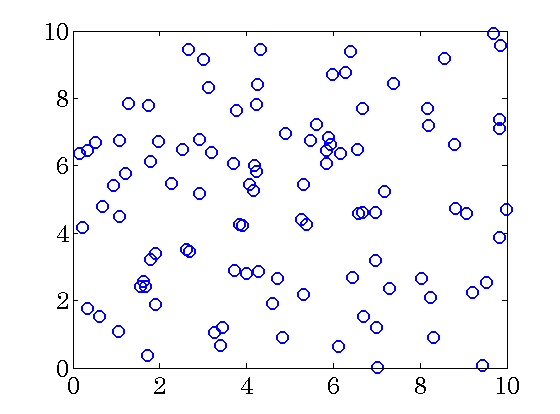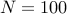nodes are randomly and uniformly distributed in a square region of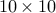units. Each node is an unstable second order linear system coupled with other nodes through the following dynamics (Motee and Jadbabaie ’08)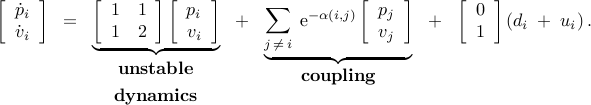The coupling between two nodesand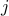is determined by the Euclidean distance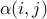between them. The performance weights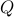and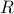are set to identity matrices.

We next present the Matlab code and the computational results obtained using lqrsp.m. In this example, we use the weighted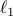norm to promote sparsity. We set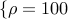,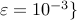and select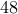logarithmically-spaced points for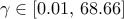.

## Matlab code

```% An example from Motee and Jadbabaie,
% "Optimal control of spatially distributed systems",
% IEEE Trans. Automat. Control,
% vol. 53, no. 7, pp. 1616–1629, 2008.

% N nodes randomly distributed in a box [0 L] x [0 L]
N = 100;
L = 10;

% or generate the random distribution of the nodes
% pos = L*rand(N,2);

% the state-space representation of subsystem i
Aii = [1 1; 1 2];
Bii = [0; 1];
n   = size(Bii,1);
Aij = eye(n);

B1  = kron(eye(N), Bii);
B2  = B1;

% construct
% (a) the Euclidean distance matrix that describes the distance
% between two systems i and j
% (b) the A-matrix of the distributed system

A = zeros(2*N,2*N);
dismat = zeros(N,N);
for i = 1:N
for j = i:N
if i == j
A( (i-1)*n + 1 : i*n, (j-1)*n + 1 : j*n ) = Aii;
else
dismat(i,j) = sqrt( norm( pos(i,:) - pos(j,:) )^2 );
dismat(j,i) = dismat(i,j);
A( (i-1)*n + 1 : i*n, (j-1)*n + 1 : j*n ) = Aij / exp( dismat(i,j) );
A( (j-1)*n + 1 : j*n, (i-1)*n + 1 : i*n ) = Aij / exp( dismat(j,i) );
end
end
end

% state and control penalty weights
Q = eye(2*N);
R = eye(N);

% compute sparse feedback gains
options = struct('method','wl1','gamval',logspace(-2,log10(68.6649),48), ...
'rho',100,'maxiter',10,'blksize',[1 1],'reweightedIter',1);

tic
solpath = lqrsp(A,B1,B2,Q,R,options);
toc
```

## Computational results

### Sparsity vs. performance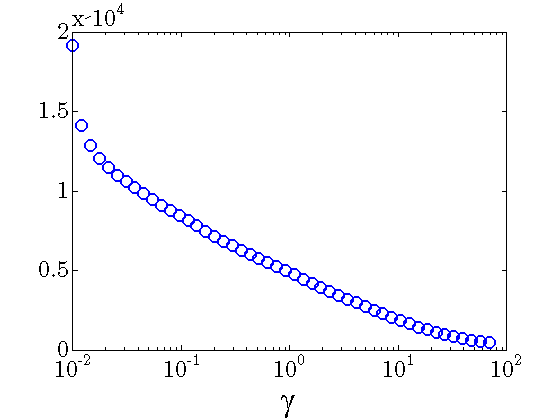Number of nonzero elements in the feedback gain decreases with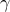.

In the absence of sparsity constraints, i.e., at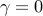, the optimal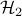controller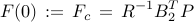is obtained from the positive definite solution of the algebraic Riccati equation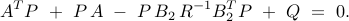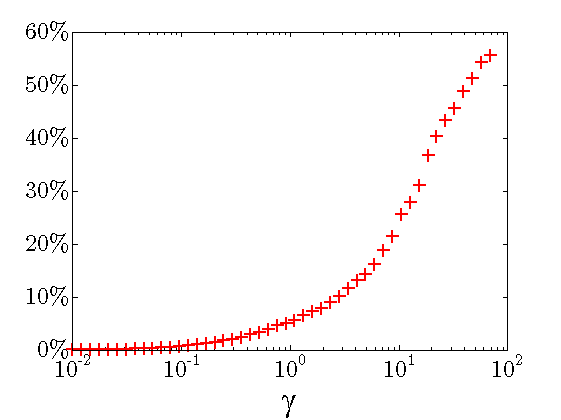Performance loss: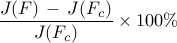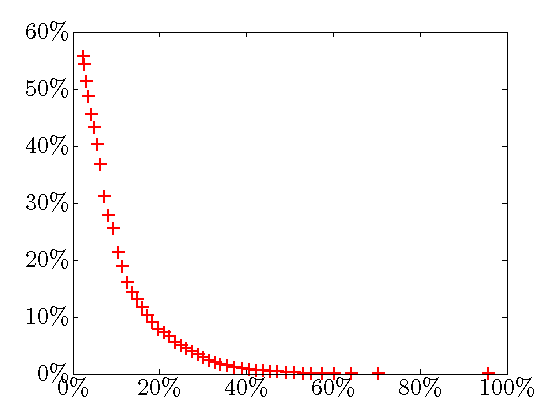Sparsity level: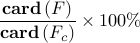Performance loss (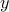-axis) vs. Sparsity level (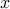-axis); As the sparsity level increases, the quadratic performance deteriorates.
Sparsity vs. performance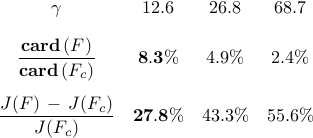The above results demonstrate that the optimal sparse feedback gain, with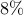of non-zero elements relative to the centralized feedback gain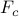, introduces performance loss of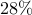compared to.

### Communication graphs of distributed controllers

Asincreases, the communication architecture of distributed controllers becomes sparser. Note that the communication graph does not have to be connected since the subsystems are

• dynamically coupled to each other;

• allowed to measure their own states.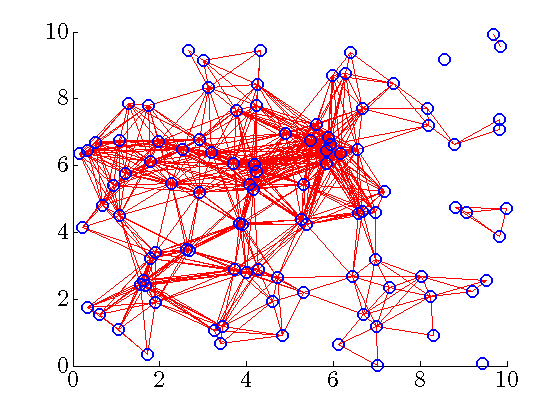Communication graph of the distributed controller for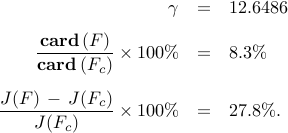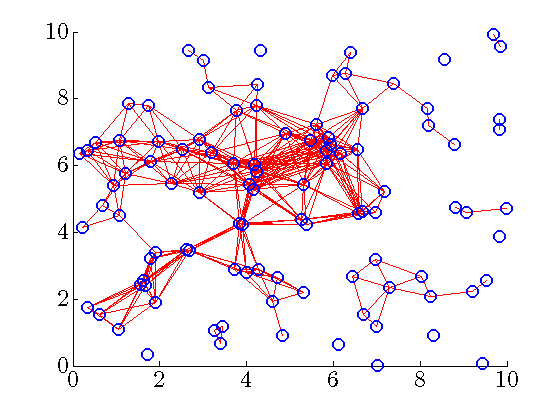Communication graph of the distributed controller for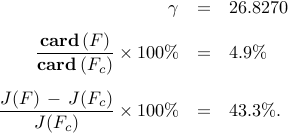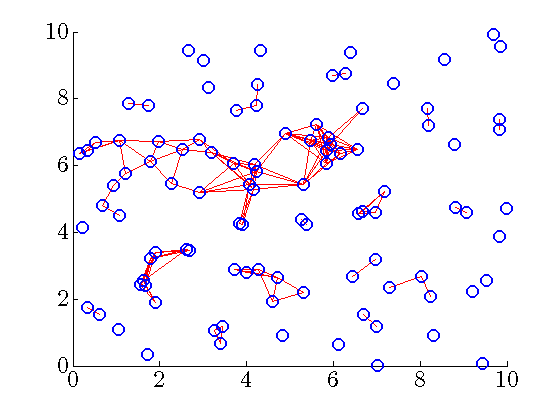Communication graph of the distributed controller for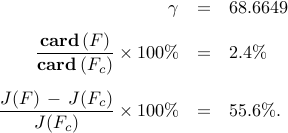### Danger of truncation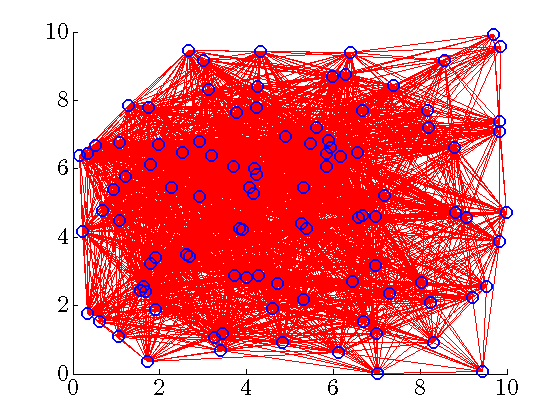Our sparsity-promoting algorithm identifies communication architectures that are difficult to guess a priori. To highlight this point, and to illustrate danger of truncation, let us consider the truncated centralized gain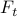,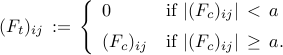The figure shows the communication graph of the truncated controller obtained by setting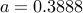. Even though the communication graph appears to be very dense (has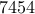nonzero elements —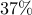relative to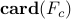), the truncated gain does not even provide the closed-loop stability.